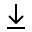MathBin Conic Member

A conic section, or simply conic, is a one-dimensional object that exists in two dimensions or higher. A conic is a curve obtained as the intersection of a cone (more precisely, a right circular conical surface) with a plane. Traditionally, the three types of conic sections are the hyperbola, the parabola, and the ellipse, where the circle is a special case of the ellipse. The type of a conic corresponds to its eccentricity, those with eccentricity less than 1 being ellipses, those with eccentricity equal to 1 being parabolas, and those with eccentricity greater than 1 being hyperbolas. In the focus-directrix definition of a conic the circle is a limiting case with eccentricity 0. In modern geometry certain degenerate cases, such as the union of two lines, are included as conics as well.

Bins in Two Dimensions Bins in Three Dimensions